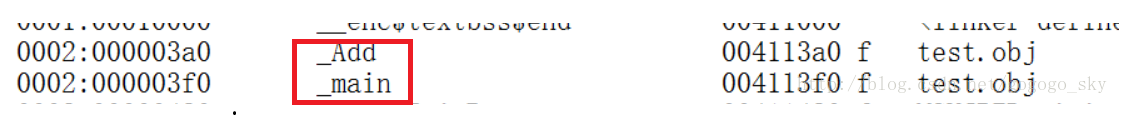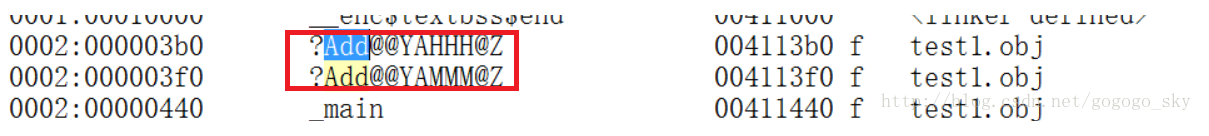# 【C++】C++中函数重载的理解

void Swap1(int* a, int* b);
void Swap2(float* a, float* b);
void Swap3(char* a, char* b);
void Swap4(double* a, double* b);

函数重载是一种特殊情况，C++允许在同一作用域中声明几个类似的同名函数这些同名函数的形参列表（参数个数，类型，顺序）必须不同，常用来处理实现功能类似数据类型不同的问题。

在C++中不仅函数可以重载，运算符也可以重载。例如：

运算符<<,>>。既可以做移位运算符，也可以做输出，输入运算符。

#include<Windows.h>
#include<iostream>
using namespace std;

{

return a + b;

}

{

return a + b;
}

{

return a + b;

}
int main()
{

cout << Add(2.22, 3.33) << endl;

system("pause");
return 0;
}

#include<iostream>
#include<Windows.h>
using namespace std;

int main()
{
int max(int a, int b, int c);
int max(int a, int b);
int a = 10;
int b = 20;
int c = 30;

cout << max(a, b, c) << endl;
cout << max(a, b) << endl;
system("pause");
return 0;
}

int max(int a, int b, int c)
{
if (b > a)
a = b;
if (c > a)
a = c;
return a;

}

int max(int a, int b)
{

return (a > b) ? a : b;
}

• 函数名称必须相同。
• 参数列表必须不同（个数不同、类型不同、参数排列顺序不同等）。
• 函数的返回类型可以相同也可以不相同。
• 仅仅返回类型不同不足以成为函数的重载。

## 二、函数重载的作用：

（1）多态：用同一个东西表示不同的形态；
（2）多态分为：
静态多态（编译时的多态）；
动态多态（运行时的多态）；
（3）函数重载是一种静态多态；

1.C语言中为什么不能支持函数重载？2.C++中函数重载底层是如何处理的？在.cpp文件中，虽然两个函数的函数名一样，但是他们在符号表中生成的名称不一样。

‘？’表示名称开始，‘？’后边是函数名“@@YA”表示参数表开始，后边的3个字符分别表示返回值类型，两个参数类型。“@Z”表示名称结束。

3.C++中能否将一个函数按照C的风格来编译？

#include<iostream>
#include<Windows.h>
using namespace std;

extern "C" int Add(int a, int b)
{

return a + b;
}
int main()
{
cout << Add(10, 20) << endl;
system("pause");
return 0;
}


©️2019 CSDN 皮肤主题: 技术黑板 设计师: CSDN官方博客